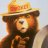# How to convert StochRSI into MTF StochRSI?

H

#### HighBredCloud

##### Well-known member
VIP
Can't seem to figure this out...Where would I put this line of code to turn regular StochRSI into MTF?

(period = aggregationPeriod)

Code:
``````#
# TD Ameritrade IP Company, Inc. (c) 2008-2020
#

declare lower;

input RSI_length = 14;
input over_bought = 80;
input over_sold = 20;
input RSI_average_type = AverageType.WILDERS;
input RSI_price = close;
input KPeriod = 14;
input DPeriod = 3;
input slowing_period = 1;
input averageType = AverageType.SIMPLE;
input showBreakoutSignals = {default "No", "On FullK", "On FullD", "On FullK & FullD"};
input aggregationPeriod = AggregationPeriod.FIFTEEN_MIN;

def RSI = RSI(price = RSI_price, length = RSI_length, averageType = RSI_average_type);

plot FullK = StochasticFull(over_bought, over_sold, KPeriod, DPeriod, RSI, RSI, RSI, slowing_period, averageType).FullK;
plot FullD = StochasticFull(over_bought, over_sold, KPeriod, DPeriod, RSI, RSI, RSI, slowing_period, averageType).FullD;
plot OverBought = over_bought;
plot OverSold = over_sold;

def upK = FullK crosses above OverSold;
def upD = FullD crosses above OverSold;
def downK = FullK crosses below OverBought;
def downD = FullD crosses below OverBought;

plot UpSignal;
plot DownSignal;
switch (showBreakoutSignals) {
case "No":
UpSignal = Double.NaN;
DownSignal = Double.NaN;
case "On FullK":
UpSignal = if upK then OverSold else Double.NaN;
DownSignal = if downK then OverBought else Double.NaN;
case "On FullD":
UpSignal = if upD then OverSold else Double.NaN;
DownSignal = if downD then OverBought else Double.NaN;
case "On FullK & FullD":
UpSignal = if upK or upD then OverSold else Double.NaN;
DownSignal = if downK or downD then OverBought else Double.NaN;
}

UpSignal.setHiding(showBreakoutSignals == showBreakoutSignals."No");
DownSignal.setHiding(showBreakoutSignals == showBreakoutSignals."No");

FullK.SetDefaultColor(GetColor(5));
FullD.SetDefaultColor(GetColor(0));
OverBought.SetDefaultColor(GetColor(1));
OverSold.SetDefaultColor(GetColor(1));
UpSignal.SetDefaultColor(Color.UPTICK);
UpSignal.SetPaintingStrategy(PaintingStrategy.ARROW_UP);
DownSignal.SetDefaultColor(Color.DOWNTICK);
DownSignal.SetPaintingStrategy(PaintingStrategy.ARROW_DOWN);``````

S

#### SparkyFlary

##### Member
The "input RSI_price = close" try changing that to a "def RSI_price = close". The close doesn't seem to like be an input.

Code:
``````#
# TD Ameritrade IP Company, Inc. (c) 2008-2020
#

declare lower;

input RSI_length = 14;
input over_bought = 80;
input over_sold = 20;
input RSI_average_type = AverageType.WILDERS;
input KPeriod = 14;
input DPeriod = 3;
input slowing_period = 1;
input averageType = AverageType.SIMPLE;
input showBreakoutSignals = {default "No", "On FullK", "On FullD", "On FullK & FullD"};
input aggregationPeriod = AggregationPeriod.FIFTEEN_MIN;

def RSI_price = close(period = aggregationPeriod);

def RSI = RSI(price = RSI_price, length = RSI_length, averageType = RSI_average_type);

plot FullK = StochasticFull(over_bought, over_sold, KPeriod, DPeriod, RSI, RSI, RSI, slowing_period, averageType).FullK;
plot FullD = StochasticFull(over_bought, over_sold, KPeriod, DPeriod, RSI, RSI, RSI, slowing_period, averageType).FullD;
plot OverBought = over_bought;
plot OverSold = over_sold;

def upK = FullK crosses above OverSold;
def upD = FullD crosses above OverSold;
def downK = FullK crosses below OverBought;
def downD = FullD crosses below OverBought;

plot UpSignal;
plot DownSignal;
switch (showBreakoutSignals) {
case "No":
UpSignal = Double.NaN;
DownSignal = Double.NaN;
case "On FullK":
UpSignal = if upK then OverSold else Double.NaN;
DownSignal = if downK then OverBought else Double.NaN;
case "On FullD":
UpSignal = if upD then OverSold else Double.NaN;
DownSignal = if downD then OverBought else Double.NaN;
case "On FullK & FullD":
UpSignal = if upK or upD then OverSold else Double.NaN;
DownSignal = if downK or downD then OverBought else Double.NaN;
}

UpSignal.setHiding(showBreakoutSignals == showBreakoutSignals."No");
DownSignal.setHiding(showBreakoutSignals == showBreakoutSignals."No");

FullK.SetDefaultColor(GetColor(5));
FullD.SetDefaultColor(GetColor(0));
OverBought.SetDefaultColor(GetColor(1));
OverSold.SetDefaultColor(GetColor(1));
UpSignal.SetDefaultColor(Color.UPTICK);
UpSignal.SetPaintingStrategy(PaintingStrategy.ARROW_UP);
DownSignal.SetDefaultColor(Color.DOWNTICK);
DownSignal.SetPaintingStrategy(PaintingStrategy.ARROW_DOWN);``````How do I convert this ATR% chart study into a scanner watchlist column? Questions 5